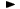ADVFN HomeHelpFinancialsKey FiguresDividend Cover

# Dividend Cover

Dividend Cover ratio, is calculated as the earnings per share-basic (EPS-basic) divided by the annual total dividend amount per share. Dividend cover expresses a company's ability to pay ordinary dividends to shareholders out of profits earned. It shows how many times the ordinary dividend is covered by the profit available and, for example, if a company pays out one quarter of its profit as dividends, then the Dividend cover ratio is four. The calculation is the following:

= EPS-basic / annual dividend per share (DPS)